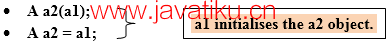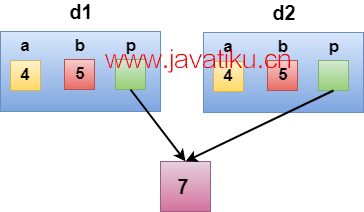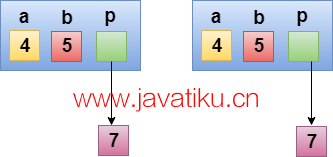# C++教程-C++拷贝构造函数## C++拷贝构造函数

### 拷贝构造函数有两种类型：

• 默认拷贝构造函数：编译器定义了默认的拷贝构造函数。如果用户未定义拷贝构造函数，编译器将提供其构造函数。
• 用户定义的构造函数：程序员定义了用户定义的构造函数。### 用户自定义拷贝构造函数的语法：

`` Class_name(const class_name &old_object); ``

``````class A
{
A(A &x) // 拷贝构造函数
{
// 拷贝构造函数
}
}``````// 拷贝构造函数的程序。

``````#include <iostream>
using namespace std;
class A
{
public:
int x;
A(int a) // 带参数的构造函数
{
x=a;
}
A(A &i) // 拷贝构造函数
{
x = i.x;
}
};
int main()
{
A a1(20); // 调用带参数的构造函数
A a2(a1); // 调用拷贝构造函数
cout<<a2.x;
return 0;
}``````

``20 ``

### 何时调用拷贝构造函数

• 当以值传递相同类类型的对象作为参数时。
• 当函数通过值返回相同类类型的对象时。

• 浅拷贝
• 深拷贝

### 浅拷贝

• 默认的拷贝构造函数只能产生浅拷贝。
• 浅拷贝被定义为通过直接复制所有成员变量的数据来创建对象的副本的过程。

``````#include <iostream>

using namespace std;

class Demo
{
int a;
int b;
int *p;
public:
Demo()
{
p=new int;
}
void setdata(int x,int y,int z)
{
a=x;
b=y;
*p=z;
}
void showdata()
{
std::cout << "a的值为: " <<a<< std::endl;
std::cout << "b的值为: " <<b<< std::endl;
std::cout << "*p的值为: " <<*p<< std::endl;
}
};
int main()
{
Demo d1;
d1.setdata(4,5,7);
Demo d2 = d1;
d2.showdata();
return 0;
}``````

``````a的值为: 4
b的值为: 5
*p的值为: 7 ``````### 深拷贝

``````#include <iostream>
using namespace std;
class Demo
{
public:
int a;
int b;
int *p;
cCopy code
Demo()
{
p=new int;
}
Demo(Demo &d)
{
a = d.a;
b = d.b;
p = new int;
*p = *(d.p);
}
void setdata(int x,int y,int z)
{
a=x;
b=y;
*p=z;
}
void showdata()
{
std::cout << "a的值为: " <<a<< std::endl;
std::cout << "b的值为: " <<b<< std::endl;
std::cout << "*p的值为: " <<*p<< std::endl;
}
};
int main()
{
Demo d1;
d1.setdata(4,5,7);
Demo d2 = d1;
d2.showdata();
return 0;
}``````

``````a的值为: 4
b的值为: 5
*p的值为: 7``````